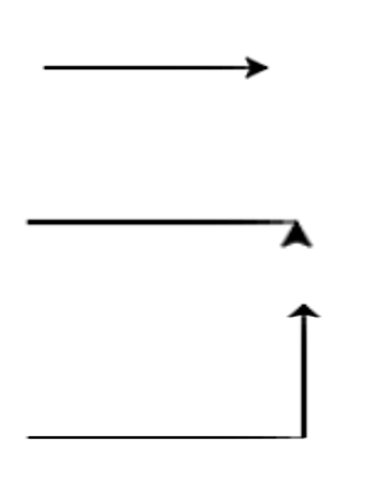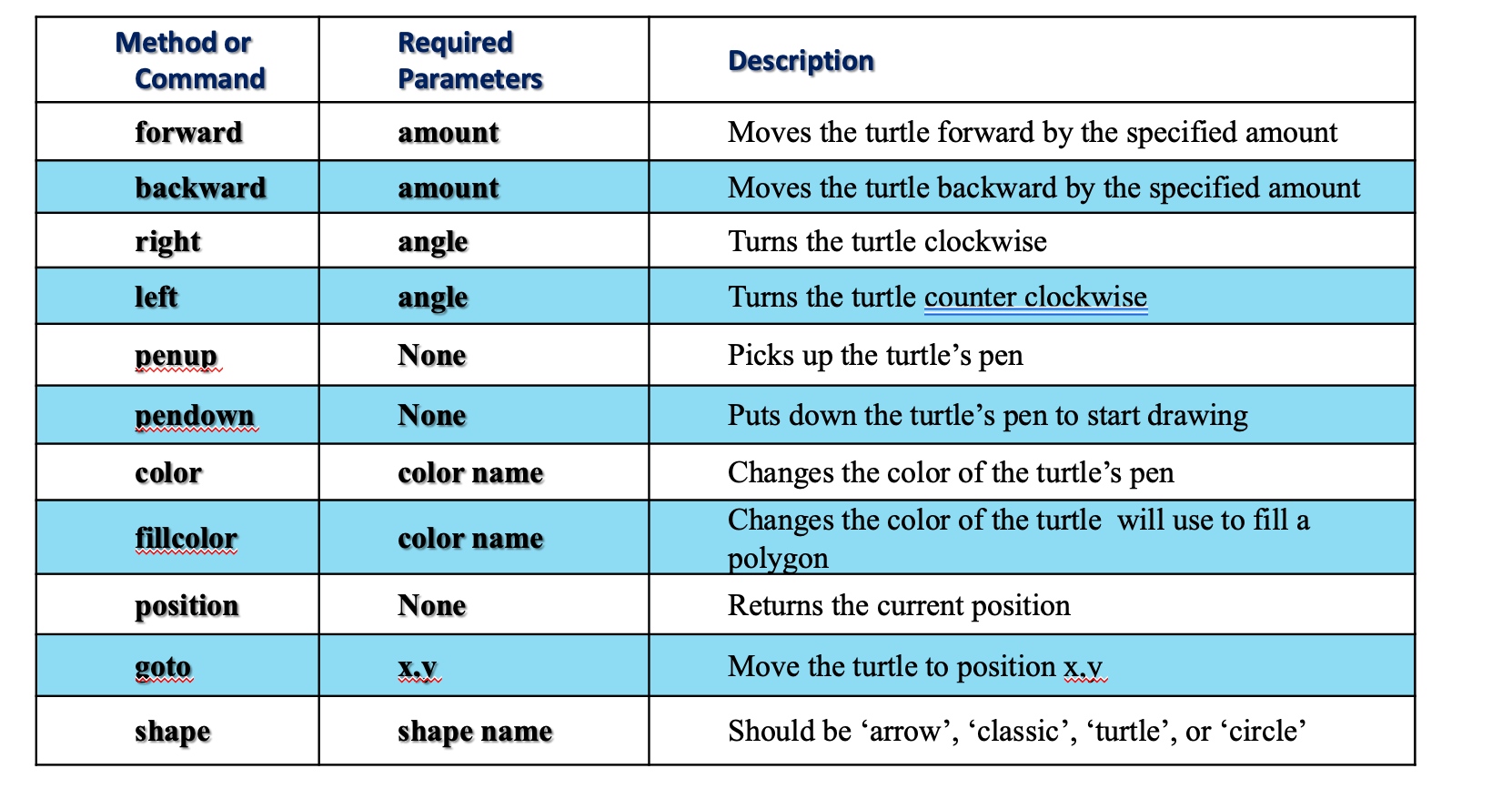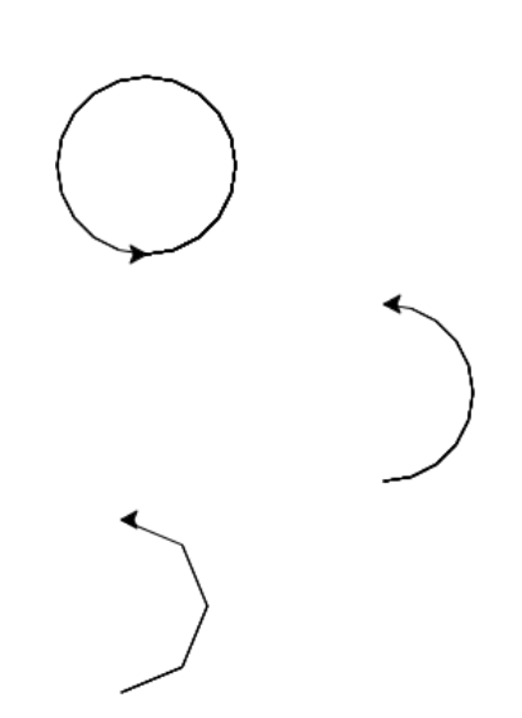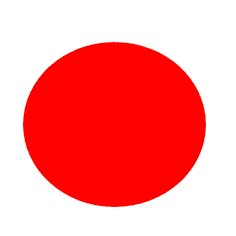# Translating Algorithm

## Translating algorithm to Python Code

How to implement?
We will use a website called https://repl.it to implement algorithms

• The turtle library has built-in commands that make it easy to draw an object.
• To access a library in Python, you need to use the keyword import.
• For example, if you want to draw a line with length 100 points, you can simply use the command forward(100).
• ```                import turtle as t
t.forward(100)
t.left(90)
t.forward(100)
```
•### Example-1

Drawing a square in Python - turtle Size of each side = 100 dots
Algorithm
• Move pen forward by 100 dots
• Change the angle to the left by 90 degrees
• Move pen forward by 100 dots
• Change the angle to the left by 90 degrees
• Move pen forward by 100 dots
• Change the angle to the left by 90 degrees
• Move pen forward by 100 dots
• Change the angle to the left by 5 degrees
• ```         Python code
1. import turtle as t
2. t.forward(100)
3. t.left(90)
4. t.forward(100)
5. t.left(90)
6. t.forward(100)
7. t.left(90)
8. t.forward(100)
9. t.left(5)
```

### Repetition

```         import turtle as t
for i in range(4):
t.forward(100)
t.left(90)
```

### Repetition using: for counter in range(start, end): [list of instructions]

This loop, repeats [list of instructions] from counter = start until end - 1
```         import turtle as t
for i in range(1,5):
t.forward(100)
t.left(90)
```

## Some turtle commands### Drawing a circle

• Syntax: turtle.circle(radius, extent = None, steps = None)
• extent – an angle – determines which part of the circle is drawn. If extent is not given, draw the entire circle. If extent is not a full circle, one endpoint of the arc is the current pen position.
• steps determines the number of steps to use
• Draw the arc in counterclockwise direction if radius is positive, otherwise in clockwise direction.

### Example of drawing a circle

```         import turtle as t
t.circle(50)
t.circle(50,180)
t.circle(50,180,4)
```### fillcolor ( colorName )

• Fills a closed shape with a color
• Should be used with begin_fill() and end_fill()
• Example:
1. t.fillcolor("red")
2. t.begin_fill()
3. t.circle( 50)
4. t.end_fill()